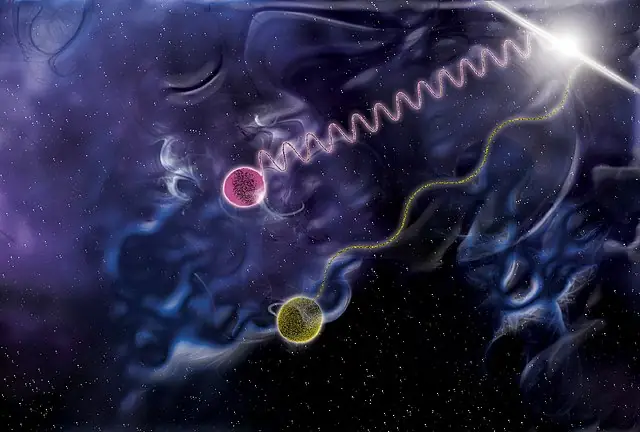# How To Calculate Energy From Wavelength Of A Photon?In this illustration, one photon (violet) carries a million times the energy of another (yellow). What happens to the energy if the wavelength is doubled?

Light, an essential component of our daily lives, carries with it energy that influences various scientific and technological applications. Knowing how to calculate energy from wavelength is crucial for understanding the behavior and properties of light in different scenarios, from harnessing solar energy to developing communication systems. In this article, we will explore the basic principles and concepts needed to calculate energy from wavelength, providing a solid foundation to better comprehend the diverse roles that light plays in science, technology, and the world around us.

## The Electromagnetic Spectrum: Understanding Wavelengths

Before diving into the calculations, let’s first explore the electromagnetic spectrum. It is the range of all possible frequencies of electromagnetic radiation, which includes visible light, infrared, ultraviolet, X-rays, and gamma rays. The wavelength of light is the distance between two consecutive points in the same phase of a wave, such as from crest to crest. Wavelengths are measured in units of length, like meters or nanometers. Understanding the relationship between energy and wavelength is crucial for various applications, from communication technology to medical imaging.

## Light as Photons: The Particle-Wave Duality

One of the groundbreaking discoveries in physics was the particle-wave duality of light. Scientists like Albert Einstein and Max Planck revealed that light behaves both as a wave and as a particle, called a photon. A photon is a discrete bundle of energy that travels through space at the speed of light. The energy of a photon is directly proportional to its frequency and inversely proportional to its wavelength.

## The Planck-Einstein Relation: Connecting Energy and Wavelength

The Planck-Einstein relation is a fundamental formula that connects the energy of a photon with its wavelength. The formula is:

E = h * c / λ

where:

• E is the energy of the photon (measured in joules)
• h is Planck’s constant (approximately 6.63 × 10^-34 Js)
• c is the speed of light (approximately 3.00 × 10^8 m/s)
• λ is the wavelength (measured in meters)

With this equation, we can calculate the energy of a photon based on its wavelength.

## Example: Calculating Energy from Wavelength

Let’s consider an example to illustrate how to use the Planck-Einstein relation. Suppose we have a green light with a wavelength of 520 nm (nanometers). First, we need to convert the wavelength to meters:

520 nm * (1 m / 10^9 nm) = 5.20 × 10^-7 m

Now, we can use the Planck-Einstein relation to calculate the energy:

E = (6.63 × 10^-34 Js) * (3.00 × 10^8 m/s) / (5.20 × 10^-7 m) = 3.82 × 10^-19 J

Thus, the energy of a photon of green light with a wavelength of 520 nm is approximately 3.82 × 10^-19 joules.

## Applications and Implications

Understanding how to calculate energy from wavelength has a wide range of applications. In telecommunications, it helps engineers design systems with optimal frequencies for data transmission. In medical imaging, it aids in selecting the appropriate wavelength for diagnostic procedures. Moreover, this knowledge has also contributed to the development of laser technology, solar panels, and even quantum computing.In April 2015 the HARPS laser frequency comb was installed on the HARPS planet-finding instrument on the ESO 3.6-metre telescope at the La Silla Observatory in Chile after completion of an intense first commissioning phase. The increase in accuracy made possible by this new installation should in future allow HARPS to be able to detect Earth-mass planets in Earth-like orbits around other stars for the first time. This picture shows a HARPS spectrum of the light wavelengths from the two laser frequency combs that that were tested.

## Conclusion

Calculating energy from wavelength is a fundamental concept in the world of light and physics. It not only helps us appreciate the beauty of the electromagnetic spectrum but also has practical applications across various scientific and technological fields. By grasping this essential relationship, we unlock the door to a deeper understanding of the world around us.